# Electrical and Electronics Engineering January 2013

UNIT -1

Q 1. (a) Find the current in the ammeter of the 2 Q. resistance branch as shown in figure 1(a) using thevenin’s theorem.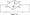(b) Compute the power dissipated in 9^ resistor by applying superposition in circuit of figure 1(b).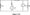OR

(a) State & explain The venin’s theorem with suitable example.

(b) Find the current through the 1Q resistor using node voltage method for the circuit shown below.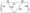UNIT – II

Q. 2 (a) A periodic voltage wave form has been shown in figure 2 (a). Determine:

(i) Frequency of wave from

(ii) Wave equation for 0 < / < 100m sec.

(iii) RMS Value.

(iv) Average Value.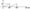V(Volt)

(b) Explain the generation of single phase AC Voltage & derive its EMF equation.

OR

Q.2 (a) Find the average value & RMS Value of a half rectified current wave as shown below.

(b) Define & determine average value & root mean square value of an alternating sinusoidal quantity.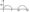UNIT-III

Q. 3  (a) Derive the EMF equation of DC machine.

(b) Explain the working principle of DC motor and compare its types.

OR

Q.3. (a) A Separately excited DC generator has an armature emf of 100V when the flux per pole is 20 mwb and the speed is 800 RPM. Calculate the generated emf with the same flux and the speed at 1500 RPM.

(b) State the basic principle of synchronous motor & briefly explain its working.

UNIT – IV

Q.4.(a) How transistor works as an amplifier? Explain its amplification phenomenon.

(b) A transistor operating in CB configuration has Ic = 2.98MA, IE = 3.0MA and Ico = 0.01MA. What current flows in the collector circuit of this transistor when connected in CE configuration with a base current of30nA ?

Q.4. (a) Sketch and explain input & output characteristics of a transistor connected in common emitter configuration.

(b) Define fi for a transistor. Derive the relationship between a and p of a transistor.          .

UNIT -V

Q.5. (a) What is the need of modulation? Compare the different types of modulation techniques used in communication.

(b) Write short notes on:

(i) Thermocouple.

(ii) Strain gauge.

OR

Q.5. (a) Define amplitude modulation & analyze frequency spectrum of AM wave.

(b) Write short notes on:

(i) R T D.